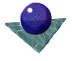Least squares estimation
of 3D poseFrom equations 1, 2 and 3 the 3D-3D pose estimation problem is to infer R and T from the sets of corresponding points. Expressed as a least squares problem, we wish to minimise,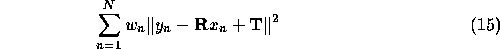To simplify this problem, we can compute the mean values (centroids) of each set of points, and translate them in space so that these lie at the origin. Then compute R, such that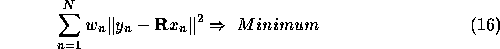To minimise this function, we first expand the left hand side of Equation 15. This requires some matrix algebra (See ref. 2,Kanatani, 93, pages 21, 106, 118, 133). For two matrices,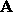and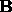,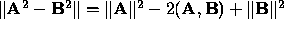. The notation,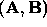denotes the Euclidean inner product of vectors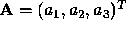and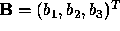which is equal to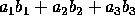. Further,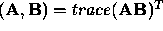.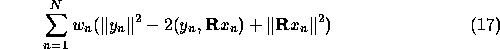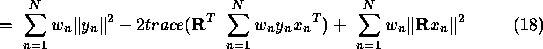Re-examining equation 18, above, the first and third terms are fixed depending on the weighting function and the point sets. Hence we wish to maximise the second term in order to minimise the whole expression. In other words,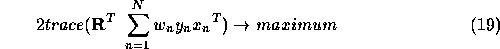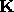is the correlation matrix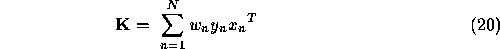Hence the problem can now be stated. Compute a rotation matrix, R, so that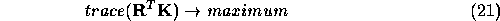Solutions to this problem can be found based on singular value decomposition, polar value decomposition or quaternions (See references Haralick et al, 1989, and Kanatani, 1993.) Based on singular value decomposition, we decompose the correlation matrix, K into the form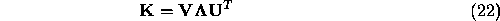whereand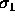,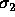and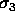are the singular values. The rank of K is equal to the number of linearly independent columns or rows of K. Since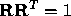, Equation 19, above, is maximised if,This gives a unique solution for the rotation matrix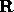provided the rank of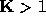and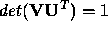, or if the rank ofand the minimum singular value is a simple root. Finally, the translation vector,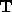is defined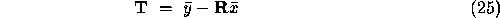Comments to: Sarah Price at ICBL.